• 高中数学圆锥曲线统一的极坐标方程课后作业理北师大选修PPT课件.pptx
• 2019_2020学年高中数学课时作业8圆锥曲线统一的极坐标方程北师大版选修4_4202004200159
• 江西省九江市实验中学高中数学 第一章 第六课时 圆锥曲线统一的极坐标方程教学案（无答案）新人教A版选修4-4
• 高考主要考查直线和圆的极坐标方程，参数方程与普通方程的互化，常见曲线的参数方程及参数方程的简单应用，以极坐标、参数方程与普通方程的互化为主要考查形式，同时考查直线与曲线位置关系等解析几何知识....各位朋友大家好，今天我们一起来看一下选修4-4所涉及到一个专题：极坐标与参数方程。
该章在高考中只考查1个大题，以解答题形式出现，占10分，属于二选一的题目.高考主要考查直线和圆的极坐标方程，参数方程与普通方程的互化，常见曲线的参数方程及参数方程的简单应用，以极坐标、参数方程与普通方程的互化为主要考查形式，同时考查直线与曲线位置关系等解析几何知识.从命题趋势来看，估计2019年高考围绕坐标方程互化，利用参数方程解决直线与圆锥曲线的综合问题，突出参数方程的优势，特别是直线与圆和椭圆。
特别说明：本专题主要适合参加2019年的高考考生，对于参加2020年及以后高考的考生，可以做一些简单的了解即可，估计2020年及以后的全国卷高考会取消该章节的考察。该章节所呈现出来的参数思想与坐标系的另一种理解，对于我们提升数学思维和拓展解决问题的思路都很有帮助，让我们一起来看一下该章节所涉及到的知识点吧。
第一讲　坐标系
1．平面直角坐标系中的坐标伸缩变换
设点P(x，y)是平面直角坐标系中的任意一点，在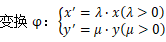的作用下，点P(x，y)对应到点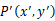，称φ为平面直角坐标系中的坐标伸缩变换，简称伸缩变换．
伸缩变换公式应用时的两个注意点
(1)曲线的伸缩变换是通过曲线上任意一点的坐标的伸缩变换实现的，解题时一定要区分变换前的点P的坐标(x，y)与变换后的点P′的坐标(X，Y)，再利用伸缩变换公式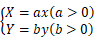建立联系．
(2)已知变换后的曲线方程f(x，y)＝0，一般都要改写为方程f(X，Y)＝0，再利用换元法确定伸缩变换公式．
2．极坐标系与点的极坐标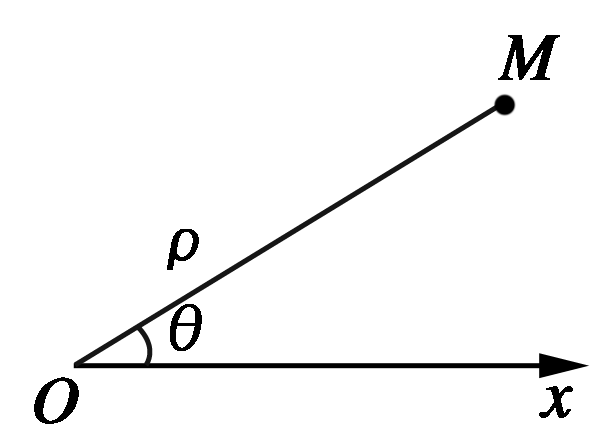(1)极坐标系：
在平面上取一个定点O，由O点出发的一条射线Ox，一个长度单位及计算角度的正方向(通常取逆时针方向)，合称为一个极坐标系，O点称为极点，Ox称为极轴，平面上任一点M的位置可以由线段OM的长度ρ和从Ox到OM的角度θ来刻画(如图)，这两个数组成的有序数对(ρ，θ)称为点M的极坐标，ρ称为极径，θ称为极角.
(2)极坐标与直角坐标的互相转化：
①互相转化的前提条件：
a．极点与坐标原点重合；
b.极轴与x轴正半轴重合，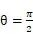的射线与y轴正半轴重合；
c.取相同的单位长度．
②互相转化公式：
设点P的直角坐标为(x，y)，它的极坐标为(ρ，θ)，则互相转化公式为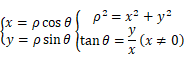
(1)极坐标与直角坐标互化公式的三个前提条件
①取直角坐标系的原点为极点．
②以x轴的非负半轴为极轴．
③两种坐标系规定相同的长度单位．
(2)直角坐标化为极坐标的关注点
①根据终边相同的角的意义，角θ的表示方法具有周期性，故点M的极坐标(ρ，θ)的形式不唯一，即一个点的极坐标有无穷多个．
当限定ρ≥0，θ∈[0,2π)时，除极点外，点M的极坐标是唯一的．
②当把点的直角坐标化为极坐标时，求极角θ应注意判断点M所在的象限(即角θ的终边的位置)，以便正确地求出角θ∈[0,2π)的值．
3．直线的极坐标方程
(1)特殊位置的直线的极坐标方程：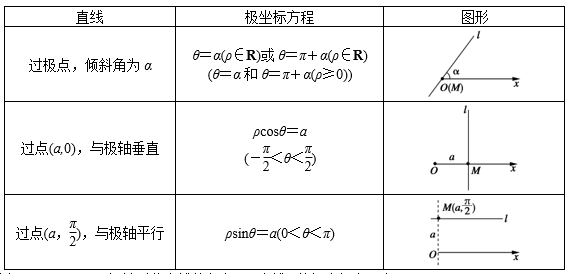(2)一般位置的直线的极坐标方程：若直线l经过点M(ρ0，θ0)，且极轴到此直线的角为α，直线l的极坐标方程为：ρsin(α－θ)＝ρ0sin(α－θ0).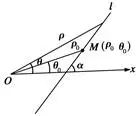4．半径为r的圆的极坐标方程
(1)特殊位置的圆的极坐标方程：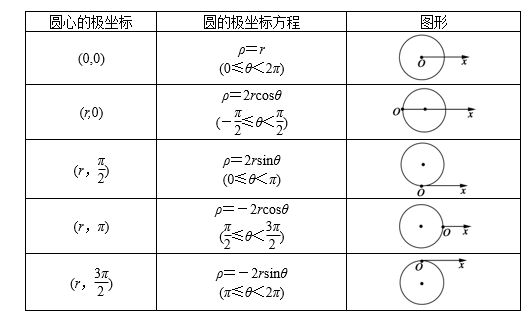(2)一般位置的圆的极坐标方程：圆心为M(ρ0，θ0)，半径为r的圆的极坐标方程为
ρ2－2ρ0ρcos(θ－θ0)＋ρ－r2＝0.
极坐标方程及其应用的类型及解题策略
(1)求极坐标方程。可在平面直角坐标系中，求出曲线方程，然后再转化为极坐标方程。
(2)求点到直线的距离。先将极坐标系下点的坐标、直线方程转化为平面直角坐标系下点的坐标、直线方程，然后利用直角坐标系中点到直线的距离公式求解。
(3)求线段的长度。先将极坐标系下的点的坐标、曲线方程转化为平面直角坐标系下的点的坐标、曲线方程，然后再求线段的长度。
求曲线的极坐标方程的步骤：
(1)建立适当的极坐标系，设P(ρ，θ)是曲线上任意一点；
(2)由曲线上的点所适合的条件，列出曲线上任意一点的极径ρ和极角θ之间的关系式；
(3)将列出的关系式进行整理、化简，得出曲线的极坐标方程．
需要注意的两点：
1.简单曲线的极坐标方程可结合极坐标系中ρ和θ的具体含义求出，也可利用极坐标方程与直角坐标方程的互化公式得出．同直角坐标方程一样，由于建系的不同，曲线的极坐标方程也会不同，在没有充分理解极坐标的前提下，可先化成直角坐标解决问题．
2．把直角坐标化为极坐标，求极角θ时，应注意判断点P所在的象限(即角θ的终边的位置)，以便正确地求出角θ.第二讲　参数方程
1．参数方程的概念
如果曲线C上任意一点P的坐标x和y都可以表示为某个变量t的函数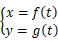反过来，对于t的每个允许值，由函数式所确定的点P(x，y)都在曲线C上，那么方程叫做曲线C的参数方程，变量t是参数．
2．圆锥曲线的参数方程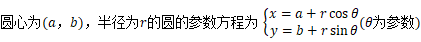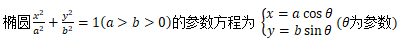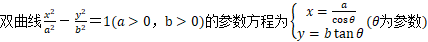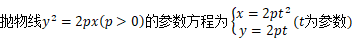3．直线的参数方程过点M(x0，y0)，倾斜角为α的直线l的参数方程为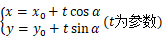其中t表示直线上以定点M0为起点，任意一点M(x，y)为终点的有向线段的数量.当t＞0时，的方向向上；当t＜0时，的方向向下；当t＝0时，M与M0重合．
将参数方程化为普通方程的方法
(1)将参数方程化为普通方程，需要根据参数方程的结构特征，选取适当的消参方法．常见的消参方法有：代入消参法、加减消参法、平方消参法等，若参数为“t”，一般直接代入消参即可．对于含三角函数的参数方程，常利用同角三角函数关系式消参．如sin2θ＋cos2θ＝1等．
(2)将参数方程化为普通方程时，要注意两种方程  的等价性，不要增解。
极坐标方程与参数方程综合问题的解题策略
(1)求交点坐标、距离、线段长。可先求出直角坐标方程，然后求解．
(2)判断位置关系。先转化为平面直角坐标方程，然后再作出判断．
(3)求参数方程与极坐标综合的问题。一般是先将方程化为直角坐标方程，利用直角坐标方程来研究问题．
思想方法—直线参数方程中参数t的几何意义
过定点M0(x0，y0)，倾斜角为α的直线的参数方程为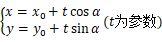通常称它为直线l的参数方程的“标准式”．其中参数t的几何意义是：|t|是直线上任一点M(x，y)到M0(x0，y0)的距离，即|M0M|＝|t|.
当0<α时，sinα>0，所以，直线l的单位方向向量e的方向总是向上．此时，若t>0，则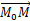的方向向上；若t<0，则的方向向下；若t＝0，则点M与点M0重合．即当点M在M0上方时，有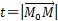；当点M在M0下方时，有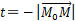该参数t经常用在直线截圆锥曲线的距离问题中，解题时通常过某定点作一直线与圆锥曲线相交于A，B两点，所求问题与定点到A，B两点的距离有关解题时主要应用定点在直线AB上，利用参数t的几何意义，结合根与系数的关系进行处理，巧妙求出问题的解．
(1)若M1、M2是直线l上的两个点，对应的参数分别为t1，t2，则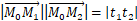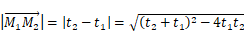(2)若线段M1M2的中点为M3，点M1、M2、M3对应的参数分别为t1、t2、t3，则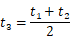(3)若直线l上的线段M1M2的中点为M0(x0，y0)，则t1＋t2＝0，t1t2＜0.
利用曲线的参数方程来求解两曲线间的最值问题非常简捷方便，是我们解决这类问题的好方法．另外，我们需要注意再将曲线的参数方程化为普通方程时，还要注意其中的x，y的取值范围，即在消去参数的过程中一定要注意普通方程与参数方程的等价性．
以上就是该专题所涉及到的常用基础知识点，大家做好整理和总结，一定要多看多思考，加油哦展开全文• 点击蓝字关注我们参数方程极坐标[导读]学科：数学 教学内容：参数方程极坐标 一、考纲要求 1.理解参数方程的概念，了解某些常用...教学内容：参数方程极坐标　一、考纲要求　1.理解参数方程的概念，了解某些...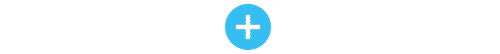点击蓝字关注我们
参数方程、极坐标
[导读]学科：数学 教学内容：参数方程、极坐标 一、考纲要求 1.理解参数方程的概念，了解某些常用参数方程中参数的几何意义或物理意义，掌握参数方 程与普通方程的互化方法.会根据所给出的参数，依据条件建立参数方程. 2.理解极坐标的概念.会正确进行点的极坐标与直角坐标的互化....
教学内容：参数方程、极坐标
一、考纲要求
1.理解参数方程的概念，了解某些常用参数方程中参数的几何意义或物理意义，掌握参数方 程与普通方程的互化方法.会根据所给出的参数，依据条件建立参数方程.
2.理解极坐标的概念.会正确进行点的极坐标与直角坐标的互化.会正确将极坐标方程化为 直角坐标方程，会根据所给条件建立直线、圆锥曲线的极坐标方程.不要求利用曲线的参数 方程或极坐标方程求两条曲线的交点.　　　　二、知识结构
1.直线的参数方程
(1)标准式  过点Po(x0,y0)，倾斜角为α的直线l(如图)的参数方程是　　　　 (t为参数)
(2)一般式  过定点P0(x0,y0)斜率k=tgα=的直线的参数方程是
(t不参数)        ②
在一般式②中，参数t不具备标准式中t的几何意义，若a2+b2=1,②即为标准式，此时， ｜ t｜表示直线上动点P到定点P0的距离；若a2+b2≠1，则动点P到定点P0的距离是
｜t｜.
直线参数方程的应用  设过点P0(x0,y0),倾斜角为α的直线l的参数方程是
(t为参数)
若P1、P2是l上的两点，它们所对应的参数分别为t1,t2，则
(1)P1、P2两点的坐标分别是
(x0+t1cosα,y0+t1sinα)
(x0+t2cosα,y0+t2sinα)；
(2)｜P1P2｜=｜t1-t2｜;
(3)线段P1P2的中点P所对应的参数为t，则　　t=　　中点P到定点P0的距离｜PP0｜=｜t｜=｜｜
(4)若P0为线段P1P2的中点，则
t1+t2=0.
2.圆锥曲线的参数方程
(1)圆  圆心在(a,b)，半径为r的圆的参数方程是
(是参数)
φ是动半径所在的直线与x轴正向的夹角，∈［0,2π］(见图)　　　　 (2)椭圆  椭圆=1(a＞b＞0)的参数方程是
(为参数)
椭圆  =1(a＞b＞0)的参数方程是
(为参数)
3.极坐标
极坐标系  在平面内取一个定点O，从O引一条射线Ox，选定一个单位长度以及计算角度的正 方向(通常取逆时针方向为正方向)，这样就建立了一个极坐标系，O点叫做极点，射线Ox叫 做极轴.
①极点；②极轴；③长度单位；④角度单位和它的正方向，构成了极坐标系的四要素，缺一 不可.
点的极坐标  设M点是平面内任意一点，用ρ表示线段OM的长度，θ表示射线Ox到OM的角度 ，那么ρ叫做M点的极径，θ叫做M点的极角，有序数对(ρ,θ)叫做M点的极坐标.(见图)　　　　极坐标和直角坐标的互化
(1)互化的前提条件
①极坐标系中的极点与直角坐标系中的原点重合；
②极轴与x轴的正半轴重合
③两种坐标系中取相同的长度单位.
(2)互化公式
三、知识点、能力点提示
(一)曲线的参数方程，参数方程与普通方程的互化
例1  在圆x2+y2-4x-2y-20=0上求两点A和B，使它们到直线4x+3y+19=0的距离分别最短和最长.
解：  将圆的方程化为参数方程：
(θ为参数)
则圆上点P坐标为(2+5cosθ，1+5sinθ)，它到所给直线的距离为d==｜4cosθ+3sinθ +6｜=5・｜(cosθ+sinθ)+｜ =5｜cos(φ-θ)+ ｜，其中cosφ=，sinφ= .故当cos(φ-θ)=1，即φ=θ时 ，d最长，这时，点A坐标为(6，4)；当cos(φ-θ)=-1,即θ=φ-π时，d最短，这时，点B坐标为(-2，2).　　　　(二)极坐标系，曲线的极坐标方程，极坐标和直角坐标的互化
说明  这部分内容自1986年以来每年都有一个小题，而且都以选择填空题出 现.
例2  极坐标方程表示的曲线C1∶ρ=f(θ),C2∶ρ=-f(π＋θ)必定是(    )
A.关于直线θ=对称            B. 关于极点对称
C.关于极轴对称                 D.同一曲线
解：因(ρ，θ)与(-ρ，π+θ)表示相同的点，
故选D.　　　　(三)综合例题赏析
例3  椭圆 (Φ是参数)的两个焦点坐标是(    )
A.(-3，5)，(-3，-3)          B.(3，3)，(3，-5)
C.(1，1)，(-7，1)          D.(7，-1)，(-1，-1)
解：化为普通方程得=1
∴a2=25,b2=9,得c2＝１６,c=4.
∴F(x-3,y+1)=F(0,±4)
∴在xOy坐标系中，两焦点坐标是(3，3)和(3，-5).
应选B.
例4  参数方程
(0 ＜θ＜2π)表示(    )
A.双曲线的一支，这支过点(1，)
B.抛物线的一部分，这部分过(1，)
C.双曲线的一支，这支过(-1，)
D.抛物线的一部分，这部分过(-1，)
解：由参数式得x2=1+sinθ=2y(x＞0)
即y=x2(x＞0).
∴应选B.
例5  曲线的参数方程为 (0≤t≤5)则曲线是(    )
A.线段          B.双曲线的一支
C.圆弧          D.射线
解  消去t2得，x-2=3(y-1)是直线
又由0≤t≤5,得2≤x≤77,故为线段
应选A.
例6  下列参数方程(t为参数)与普通方程x2-y=0表示同 一曲线的方程是(    )
A.                  B.
C.           D.
解：普通方程x2-y中的x∈R，y≥0，A.中x=｜t｜≥0，B. 中x=cost∈〔-1,1〕，故排除A.和B.
C.中y==ctg 2t==，即x2y=1，故排除C.
∴应选D.
例7  曲线的极坐标方程ρ=４sinθ化 成直角坐标方程为(    )
A.x2+(y+2)2=4          B.x2+(y-2)2=4
C.(x-2)2+y2=4          D.(x+2)2+y2=4
解：将ρ=，sinθ=代入ρ=4sinθ，得
x2+y2=4y，即x2+(y-2)2=4.
∴应选B.
例8  极坐标ρ=cos(-θ)表示的曲线是(    )
A.双曲线      B.椭圆      C.抛物线      D.圆
解：原极坐标方程化为ρ=(cosθ+sin θ)；ρ2=ρcosθ+ρsinθ，
∴普通方程为 (x2+y2)=x+y，表示圆.
应选D.
例9  在极坐标系中，与圆ρ=4sinθ相切 的条直线的方程是(    )
A.ρsinθ=2           B.ρcos θ=2
C.ρcosθ=-2          D.ρcosθ=-4
解：如图.　　　　⊙C的极坐标方程为ρ=4sinθ，CO⊥OX,OA为直径，｜OA｜=4,l和圆相切，l 交极轴于B(2，0)点P(ρ,θ)为l上任意一点，则有
cosθ=，得ρcosθ=2，
∴应选B.
例10  极坐标方程4sin2θ=3表示曲线是 (    )
A.两条射线          B.两条相交直线
C.圆                D.抛物线
解：由4sin2θ=3,得4・＝3,即y2=3 x2，y=±x,它表示两相交直线.
∴应选B.
【同步达纲练习】
(一)选择题
1.点P的直角坐标为(1，-)，则点P的极坐标为(    )
A.(2，)          B.(2，)         C.(2，-)          D.(-2，-)
2.直线：3x-4y-9=0与圆：，(θ为参数)的位置关系是(    )
A.相切                B.相离
C.直线过圆心          D.相交但直线不过圆心
3.若(x，y)与(ρ，θ)(ρ∈R)分别是点M的直角坐标和极坐标，t表示参数，则下列各组曲 线：①θ=和sinθ=；②θ=和tgθ=，③ρ2-9=0和ρ= 3；④和.
其中表示相同曲线的组数为(    )
A.1      B.2
C.3      D.4
4.设M(ρ1，θ1)，N(ρ2，θ2)两点的极坐标同时满足下列关系：ρ1+ρ2=0 ，θ1+θ2=0，则M，N两点位置关系是(    )
A.重合          B.关于极点对称
C.关于直线θ=          D.关于极轴对称
5.实数x,y，θ满足x+yi=(cosθ+isinθ)(3cosθ+isinθ),当θ
变化时，点(x,y)的轨迹是(    )
A.椭圆      B.双曲线      C.抛物线      D.圆
6.经过点M(1，5)且倾斜角为的直线，以定点M到动 点P的位移t为参数的参数方程是(    )
A.                B.
C.                D.
7.将参数方程(m是参数，ab≠0)化为普通方程是(    )
A. =1(x≠a)            B. =1(x≠-a )
C. =1(x≠a)            D. =1(x≠-a )
8.把极坐标方程ρ=2sin(+θ)化为直角坐标方程为(    )
A.(x-)2+(y-)2=1                B.y2=2(x-)
C.(x-)(y-)=0                  D.＝1
9.参数方程 (t为参数)所表示的曲线是 (    )
A.一条射线          B.两条射线
C.一条直线          D.两条直线
10.双曲线 (θ为参数)的渐近线方程为(    )
A.y-1=±(x+2)          B.y=±x
C.y-1=±2(x+2)          D.y+1=±2(x-2)
11.直线(t为参数)与圆(θ为参数)相切，则直线的倾斜角为(    )
A.或          B. 或       C. 或          D.- 或-
12.已知曲线(t为参数)上的点M，N对应的参数分别为t1，t2，且t1+t2=0，那么M，N间的距离为(    )
A.2p(t1+t2)               B.2p(t21+t22)
C.│2p(t1-t2)│           D.2p(t1-t2)2
13.若点P(x，y)在单位圆上以角速度ω按逆时针方向运动，点M(-2xy，y2-x2)也在单位 圆上运动，其运动规律是(    )
A.角速度ω，顺时针方向    B.角速度ω，逆时针方向
C.角速度2ω,顺时针方向  D.角速度2ω，逆时针方向
14.已知过曲线 (θ为参数，且0≤θ≤π)上一点P 与原点O的直线PO的倾斜角为，则P点坐标是(    )
A.(3，4)                     B.(，2)
C.(-3，-4)                   D.(，)
15.直线ρ=与直线l关于 直线θ=(ρ∈R)对称，则l的方程是(    )
A.ρ=            B.ρ=
C.ρ=            D.ρ=　　　　(二)填空题
16.双曲线  的中心坐标是                 .
17.参数方程(θ为参数)化成普通方程为            .
18.极坐标方程ρcos(θ-)=1的直角坐标方程是              .
19.抛物线y2=2px(p＞0)的一条过焦点的弦被焦点分成m、n长的两段，则=         .　　　　(三)解答题
20.设椭圆(θ为参数) 上一点P，若点P在第一象限，且∠xOP=，求点P的坐 标.
21.曲线C的方程为 (p＞0，t为参数)，当t∈［-1，2］时 ，曲线C的端点为A，B，设F是曲线C的焦点，且S△AFB=14，求P的值.
22.已知过点P(1，-2)，倾斜角为的直线l和抛物线x2=y+m
(1)m取何值时，直线l和抛物线交于两点?　　　　(2)m取何值时，直线l被抛物线截下的线段长为.　　　　23.如果椭圆的右焦点和右顶点的分别是双曲线 (θ 为参数)的左焦点和左顶点，且焦点到相应的准线的距离为，求这椭圆上的点到双曲线渐近线的最短距离.　　　　24.A，B为椭圆=1，(a＞b＞0) 上的两点，且OA⊥OB，求△AOB的面积的最大值和最小值.　　　　25.坐标平面上有动点P(cos2t+sin2t,-cos2t+sin2t),Q(cost-sint,cost+sint),t∈［0,π］,当t变化时：
(1)求P，Q两动点的轨迹；　　　　(2)当｜PQ｜＝时，求t的值.
参考答案
【同步达纲练习】
(一)1.C  2.D  3.C  4.C  5.D  6.A  7.A  8.A  9.B  10.C  11.A  12.C   13.C  14.D  15.D
(二)16.(2，-1)；17.y2=-2(x-),(x≤);18.x+y-z=0；19.
(三)20.(，)；21.；
22.(1)m＞,(2)m=3；23.(27-3)；24.Smax=，smin=;
25.(1)P点轨迹为圆x2+y2=2,Q点的轨迹为半径圆x2+y2=4(y≥0)，(2)t=或t=.


展开全文•   在圆锥曲线1中介绍了，圆锥曲线在平面直角坐标系下的统一方程。统一方程的好处是各参数具有强烈的几何意义。统一方程的不足是有的圆锥曲线其不能表达（比如圆），而且在采用5点法，解统一方程的时候也不是那么好...
圆锥曲线2_方程表示

目录
圆锥曲线2_方程表示1 圆锥曲线的一般方程1.1 一般方程的形式1.2 一般方程所能表达的曲线1.3 用5点法解一般方程
2 圆锥曲线的参式方程2.1 圆的参式表达2.2 椭圆的参式表达2.3 双曲线的参式表达2.4 抛物线的参式表达
3 圆锥曲线的极坐标方程3.1 圆的极坐标方程3.2 椭圆、双曲线、抛物线统一极坐标形式

1 圆锥曲线的一般方程
在圆锥曲线1中介绍了，圆锥曲线在平面直角坐标系下的统一方程。统一方程的好处是各参数具有强烈的几何意义。统一方程的不足是有的圆锥曲线其不能表达（比如圆），而且在采用5点法，解统一方程的时候也不是那么好解。   由此我们要寻找一种更具包容性的方程，使得其能够表达各种圆锥曲线。在平面直角坐标系下，这样的方程都是二次方程，这也是为什么圆锥曲线被称为二次曲线的原因。
1.1 一般方程的形式
一般方程：

a

x

2

+

b

y

2

+

c

x

y

+

d

x

+

e

y

+

f

=

0

ax^2+by^2+cxy+dx+ey+f=0

其中

x

,

y

x,y

为变量；

a

,

b

,

c

,

d

,

e

,

f

a,b,c,d,e,f

为实参量，且要求

a

,

b

,

c

a,b,c

不全为零。

说明：这个方程是一个二元二次方程，根据系数的不同可以表示所有的二次曲线，圆锥曲线是二次曲线，所以这个方程能表示所有的圆锥曲线。

显然圆锥曲线的标准方程稍加变形就能得到一个一般方程形式。 比如椭圆标准方程

x

2

a

2

+

y

2

b

2

=

1

\frac{x^2}{a^2}+\frac{y^2}{b^2}=1

，变形得到一般方程形式

b

2

x

2

+

a

2

y

2

−

a

2

b

2

=

0

b^2x^2+a^2y^2-a^2b^2=0

。

注意：一般方程的参数同时乘以一个不为零的实数，改方程所表达的曲线不变。

1.2 一般方程所能表达的曲线
1、可以表达一点 比如

x

2

+

y

2

=

0

x^2+y^2=0

就表示

(

0

,

0

)

(0,0)

点。 2、可以表达一线

(

x

+

y

+

1

)

2

=

0

(x+y+1)^2=0

，就表达一条直线

x

+

y

+

1

=

0

x+y+1=0

3、可以表达两条平行直线

(

x

+

y

+

1

)

(

x

+

y

+

2

)

=

0

(x+y+1)(x+y+2)=0

4、可以表达两条相交直线

(

x

+

y

+

1

)

(

2

x

+

y

+

1

)

=

0

(x+y+1)(2x+y+1)=0

5、可以表达各圆锥曲线
1.3 用5点法解一般方程
将5个点的坐标依次代入一般方程，就能够得到一个线性方程组，那么求解一般方程就转化为了解这个线性方程组。 假设5个点各不相同，那么有如下结论： 1、如果5点中任意三点不共线，那么会得出一个圆锥曲线方程的解； 2、如果只有三点共线（即任取四个点都不共线），那么是两条相交直线。 3、如果只有四点共线，那么可以得出一条确定的直线和过另一点的任意直线。 4、如果五点共线，那么得出一条确定直线和另一任意直线。

说明：一般方程确实有非常好的表达力，但是其几何意义不直观，并不能从一般方程直接寻找到圆锥曲线的几何量。一般方程可以通过坐标变换来化简，这留待以后讨论。

2 圆锥曲线的参式方程
一条曲线一般可以有无数种参式表达，圆锥曲线也不例外。下面选取的圆锥曲线的参式表达中的参数都具有较为明确的几何意义。
2.1 圆的参式表达
三角函数形式：

{

x

−

x

0

=

r

⋅

c

o

s

θ

y

−

y

0

=

r

⋅

s

i

n

θ

\left\{ \begin{matrix} x - x_0=r \cdot cos\theta \\ y - y_0=r \cdot sin\theta \end{matrix} \right.

其中

(

x

0

,

y

0

)

(x_0,y_0)

为圆心坐标，

r

r

表示圆的半径，

θ

\theta

表示x轴正向到连接圆心和点

(

x

,

y

)

(x,y)

的射线的角度（用弧度制表示）。
下图为圆心在原点，半径为

r

r

的圆的参数表示图：说明：用这样的参数表示圆，通常是方便的。比如需要求解一些距离问题的时候用这个方法，利用三角函数的性质能很好的解决问题。

有理形式：

{

x

−

x

0

=

r

⋅

t

2

−

1

t

2

+

1

y

−

y

0

=

r

⋅

2

t

t

2

+

1

\left\{ \begin{matrix} x - x_0=r \cdot \frac{t^2-1}{t^2+1} \\ \\ y - y_0=r \cdot \frac{2t}{t^2+1} \end{matrix} \right.

其中

(

x

0

,

y

0

)

(x_0,y_0)

为圆心坐标，

r

r

表示圆的半径，

t

t

为参数。
单位圆的参式表示为：

{

x

=

t

2

−

1

t

2

+

1

y

=

2

t

t

2

+

1

\left\{ \begin{matrix} x = \frac{t^2-1}{t^2+1} \\ \\ y = \frac{2t}{t^2+1} \end{matrix} \right.

用这样的参式表达，可以刻画圆上的有理点。

注意：这样的参数表达形式不能表达

(

1

,

0

)

(1,0)

点。

2.2 椭圆的参式表达
设椭圆的标准方程为：

x

2

/

a

2

+

y

2

/

b

2

=

1

x^2/a^2+y^2/b^2=1

，那么可以用一个称之为离心角的参数来参式的表达椭圆。 设离心角为

ϕ

\phi

，其中

ϕ

∈

[

0

,

2

π

)

\phi \in [0,2\pi)

，则椭圆可参式表达如下

{

x

=

a

⋅

c

o

s

ϕ

y

=

b

⋅

s

i

n

ϕ

\left\{ \begin{matrix} x = a \cdot cos\phi \\ y = b \cdot sin\phi \end{matrix} \right.

几何解释： 设

M

(

x

,

y

)

M(x,y)

为椭圆上一点椭圆的参数表达形式与圆的类似。
2.3 双曲线的参式表达
双曲线与椭圆相似同样可以利用离心角的概念，来进行参式表达。
设双曲线的标准方程为

x

2

/

a

2

−

y

2

/

b

2

=

1

x^2/a^2-y^2/b^2=1

设离心角为

ϕ

\phi

，其中

ϕ

∈

（

−

π

/

2

,

π

/

2

)

∪

(

π

/

2

,

3

π

/

2

)

\phi \in （-\pi/2,\pi/2) \cup (\pi/2,3\pi/2)

，则双曲线可参式表达如下

{

x

=

a

⋅

s

e

c

ϕ

y

=

b

⋅

t

a

n

ϕ

\left\{ \begin{matrix} x = a \cdot sec\phi \\ y = b \cdot tan\phi \end{matrix} \right.

实际上，这一表达形式利用了三角恒等式

1

+

t

a

n

2

ϕ

=

s

e

c

2

ϕ

1+{tan^2}\phi=sec^2\phi

几何解释： 设

M

(

x

,

y

)

M(x,y)

为双曲线上一点说明：1、用双曲函数的形式来表达双曲线。 双曲线的右半支：

{

x

=

a

⋅

c

o

s

h

(

t

)

y

=

b

⋅

s

i

n

h

(

t

)

\left\{ \begin{matrix} x = a \cdot cosh(t) \\ y = b \cdot sinh(t) \end{matrix} \right.

双曲线的左半支：

{

x

=

−

a

⋅

c

o

s

h

(

t

)

y

=

b

⋅

s

i

n

h

(

t

)

\left\{ \begin{matrix} x = -a \cdot cosh(t) \\ y = b \cdot sinh(t) \end{matrix} \right.

其中

t

t

为参数，取值为任意实数。 2、用有理函数来表达双曲线。

{

x

=

a

2

(

t

+

1

/

t

)

y

=

b

2

(

t

−

1

/

t

)

\left\{ \begin{matrix} x = \frac a2 (t+1/t) \\ \\ y = \frac b2 (t-1/t) \end{matrix} \right.

其中

t

∈

R

a

n

d

t

≠

0

。

2.4 抛物线的参式表达
考虑抛物线

y

2

=

2

p

x

y^2=2px

上一点

M

(

x

,

y

)

M(x,y)

，该点与原点连线的倾斜角为

α

\alpha

，如图那么就有

y

/

x

=

t

a

n

α

y/x=tan\alpha

，联立标准方程，和该方程可以得到

{

x

=

2

p

t

a

n

2

α

y

=

2

p

t

a

n

α

\left\{ \begin{matrix} x = \frac{2p}{tan^2\alpha} \\ \\ y = \frac{2p}{tan\alpha} \end{matrix} \right.

其中

α

∈

(

−

π

/

2

,

π

/

2

)

∧

α

≠

0

\alpha \in(-\pi/2,\pi/2) \wedge\alpha\ne0

。 这个参数方程不能表示原点。 只需令

t

=

1

/

t

a

n

α

t=1/tan\alpha

，那么就可以得出抛物线的一个参式表达

{

x

=

2

p

t

2

y

=

2

p

t

\large\left\{ \begin{matrix} x = 2pt^2 \\ y = 2pt \end{matrix} \right.

其中参数

t

t

的意义为

(

x

,

y

)

(x,y)

点到顶点连线斜率的倒数。当然

t

t

稍加扩展，当

t

=

0

t=0

时，就表示原点。由此可以规定

t

∈

R

t\in \mathbb R

参式表达的说明：如果将参数看作时间，那么参数方程表达了平面（空间）中点的运动过程。而一般的方程表达了这个运动过程的轨迹（像）。因此参数方程比一般方程有更多的信息，按照上述假设，参数方程完整的描述了运动过程，而一般方程只记录了运动过程所留下的轨迹。通过一般方程不可能知道运动的快慢等信息，但是通过参数方程却可以。由此可以有不同的参数选择，就好像在相同的运动轨迹上可以有不同的分段速度。

3 圆锥曲线的极坐标方程
用极坐标方程来描述圆锥曲线，可能是一种更接近物理的表示方式。这种表示方法在描述天体运动方面具有使得计算更加简单的优点。可能最早的坐标系统就是极坐标系统，人类在研究天问的时候使用的就是极坐标系统。这被称为"量天之学"。
直角坐标与极坐标互化 设极坐标平面上一点

M

M

的坐标为

(

ρ

,

θ

)

(\rho,\theta)

。 选择极点为原点，选择极轴为x正半轴，建立右手系直角坐标系。则极坐标和直角坐标之间有如下对应关系 1、极坐标转化为直角坐标

x

=

ρ

c

o

s

θ

y

=

ρ

s

i

n

θ

x=\rho cos\theta\\y=\rho sin\theta

2、直角坐标转化为极坐标

ρ

2

=

x

2

+

y

2

t

a

n

θ

=

y

x

\rho^2=x^2+y^2 \\ tan\theta=\frac yx

要具体的确定

θ

\theta

，可以根据

c

o

s

θ

=

x

/

x

2

+

y

2

,

s

i

n

θ

=

y

/

x

2

+

y

2

cos\theta=x/\sqrt{x^2+y^2},sin\theta=y/\sqrt{x^2+y^2}

来确定。

说明：

ρ

>

0

\rho>0

，则

(

ρ

,

θ

)

(\rho,\theta)

表示的点是明确的，若

ρ

<

0

\rho<0

，则约定

(

ρ

,

θ

)

(\rho,\theta)

与

(

−

ρ

,

θ

+

π

)

(-\rho,\theta+\pi)

表示同一个点。极点是唯一不定义角度的点。

3.1 圆的极坐标方程
一般形式：

ρ

2

−

2

ρ

(

a

⋅

c

o

s

θ

+

b

⋅

s

i

n

θ

)

+

c

=

0

\rho^2-2\rho(a\cdot cos\theta+b\cdot sin\theta)+c=0

其中

c

<

a

2

+

b

2

c<a^2+b^2

，

(

a

,

b

)

(a,b)

为圆心。 这个方程只需将圆的标准方程的直角坐标形式，转化为极坐标形式即可得到。 圆心为极点 那么此时就简化为

ρ

=

r

\rho=r

其中

r

r

为圆的半径。
3.2 椭圆、双曲线、抛物线统一极坐标形式
选择上述曲线的一个焦点为极点，以该焦点的到对应准线的垂足与该焦点的射线为极轴，那么可以得到上述曲线的极坐标方程为：

ρ

=

e

p

1

−

e

⋅

c

o

s

θ

\rho=\frac{ep}{1-e\cdot cos\theta}

其中

p

p

为交准距，

e

e

为离心率。证明：

M

M

到准线的距离为

ρ

⋅

c

o

s

θ

+

p

\rho\cdot cos\theta+p

因此根据焦点准线定义有，

ρ

ρ

⋅

c

o

s

θ

+

p

=

e

\frac{\rho}{\rho\cdot cos\theta+p}=e

变形后即得到结论。

说明：上述形式也可扩大到圆，圆的

e

=

0

,

p

=

∞

e=0,p=\infty

，只需设

e

p

=

r

ep=r

，即可表示极点为圆心的圆。 若将上述圆锥曲线绕极点旋转

α

\alpha

，则得到图形的极坐标方程为

ρ

=

e

p

1

−

e

⋅

c

o

s

(

θ

−

α

)

\rho=\frac{ep}{1-e\cdot cos(\theta-\alpha)}极坐标方程应用的一个例子：从牛顿第二定律到开普勒三定律

【参考条目】

《解析几何》尤承业《高等几何》梅向明

展开全文线性代数
• 校理说明：此文档原整理自贴吧的帖子，...圆锥曲线的一般方程 $Ax^2+By^2+Cxy+Dx+Ey+F=0$ 体现了圆锥曲线的普遍性质，但同时也包含了其退化形式，如圆、直线等。这里我们所要做的，是用能够体现圆锥曲线的三种形式...


校理说明：此文档原整理自贴吧的帖子，作者是实验室之殇（贴吧用户）。现在查找来源时，原帖已搜不到，但可以搜到作者自己在百度文库上传的文档，内容相同（补了一张图）。特此说明。内容有待核实，改日补注。

圆锥曲线的一般方程$Ax^2+By^2+Cxy+Dx+Ey+F=0$ 体现了圆锥曲线的普遍性质，但同时也包含了其退化形式，如圆、直线等。这里我们所要做的，是用能够体现圆锥曲线的三种形式（椭圆、双曲线、抛物线）的特征的参数（离心率 、焦点、焦准距、倾斜角）在平面内表示出任意的圆锥曲线。
首先，需要用到圆锥曲线在极坐标中的标准方程$\rho=\frac{e\rho}{1-e\cos\theta}\quad(e>0,p>0)$ 这个方程表示一个轴所在直线与极轴所在直线重合的圆锥曲线。其中极点为抛物线焦点，或椭圆左焦点，或抛物线右焦点。
这里我们规定其轴的方向向量$$\vec{n}$$，方向向右（即极轴的正方向），方便后文的解释说明。
现在将方程对应的曲线绕极点逆时针旋转$$\alpha$$角（$$0\leq\alpha<2\pi$$），此时方程变为$\rho=\frac{ep}{1-e\cos(\theta-\alpha)}$ 与极轴的夹角对应为$$\alpha$$。展开方程，化简为$\rho(1-e\cos\theta\cos\alpha-e\sin\theta\sin\alpha=ep$ 以极轴端点为原点，极轴为$$x$$轴方向，建立平面直角坐标系，则有$\begin{cases}\rho\cos\theta=x\\\rho\sin\theta=y\end{cases}$ 代入方程，化简，得到如下方程：$e|x\cos\alpha+y\sin\alpha+p|-\sqrt{x^2+y^2}=0$ 横向平移$$g$$个单位，纵向平移$$h$$个单位，使圆锥曲线焦点从$$(0,0)$$平移到$$(g,h)$$，对应方程为：$e|(x-g)\cos\alpha+(y-h)\sin\alpha+p|=\sqrt{(x-g)^2+(y-h)^2}$
以上所得方程即为圆锥曲线在平面内的统一方程（以$$e$$为离心率，$$p$$为焦准距）。
当$$e>1$$时，表示以$$F(g,h)$$为一个焦点，$$\alpha$$为$$\vec{n}$$与极轴所夹角的双曲线。当$$e=1$$时，表示以$$F(g,h)$$为焦点，$$\alpha$$为$$\vec{n}$$与极轴所夹角的抛物线。当$$e<1$$时，表示以$$F(g,h)$$为一个焦点，$$\alpha$$为$$\vec{n}$$与极轴所夹角的椭圆。
同时，我们也可看到，当$$e=0$$时，方程表示点$$F(g,h)$$。这是圆锥曲线的一种退化形式。
分析这个方程，可以发现仅有五个参数$$(e,p,\alpha,g,h)$$，就可以在平面内表示任意一个圆锥曲线，这恰能说明平面内五点可以确定一个圆锥曲线。（不包含其退化形式）
此外，根据这个方程还可以推导出其他相关量。如$$F(g,h)$$对应的准线方程$\frac x{\tan\alpha}+y+p\sqrt{1+\frac1{\tan\alpha}}-\frac g{\tan\alpha}-h=0$$$\vec{n}$$所在直线方程：$(x-g)\tan\alpha=y-h.$

转载于:https://www.cnblogs.com/xjtu-blacksmith/articles/conics.html
展开全文• 极坐标其实很简单，但...目 录—、极坐标的基本概念二、极坐标和直角坐标的转换三、直角坐标系下的曲线方程转换为极坐标系下的曲线方程 (1）圆心在原点的圆: (2）圆心过原点的椭圆方程: (3）直线四、对称中心不在原...
• 文章目录椭圆双曲线抛物线极坐标方程 作为让高中生心脏骤停的四个字，对于高考之后的人来说可谓刻骨铭心，所以定义不再赘述，直接撸图，其标准方程分别为 椭圆 双曲线 抛物线 x2a+y2b=1\frac{x^2}{a}+\frac{...python matplotlib animation
• 1. 极坐标系 2. 对数螺旋线 3. 曲线的极坐标表示 4. 圆锥曲线的几何定义
• 前言读本文前，你应该有基本的圆锥曲线知识，能够应对中等难度的题目；...极坐标PS：写这篇文章的时候我还没学极坐标和参数方程，所以难免有不太规范的地方（比如 我全都写成了 ），请多多包涵。极...
• 圆锥曲线离心率的极坐标形式6.1 抛物线离心率的极坐标形式6.2 椭圆离心率的极坐标形式6.3 直线的极坐标形式6.4 圆的极坐标形式 圆锥曲线 1.各个圆锥曲线来源 2.抛物线定义 抛物线是由平面上所有点与平面上某一定点...椭圆
• 平面曲线弧长（代数方程，参数方程，极坐标方程） 旋转曲面面积 旋转体体积 拉格朗日乘数法求最值； 几何平均数,代数平均数： 算术平均数（a+b）/2，不仅体现数字上的关系，而且体现将两个线段的和作为一个线段...
• 应用已导出的磁各向异性介质中的毕奥-萨伐尔定律的极坐标形式,求出用极坐标方程γ=γ(θ)表示的圆锥曲线焦点的磁场,解决了用定律的笛卡儿坐标形式难以求解的问题.
• ## 圆锥曲线总结

千次阅读 2020-03-11 12:07:52
过定点(x0,y0)(x_0,y_0)(x0​,y0​)，作圆锥曲线的两条切线，连接切点构成的弦叫切点弦。(也叫(x0,y0)(x_0,y_0)(x0​,y0​)的极线) 2.解析式 对于圆(x−a)2+(x−b)2=r2(x-a)^2+(x-b)^2=r^2(x−a)2+(x−b)2=r2，切点...
• 至于，超越光速产生时间倒流（时间变成虚数，在数轴旋转了180度），这只是数学上的对称性（有正就有负），所形成的方程求解，并不一定对应着物理上的客观存在。而在现实中，也没有任何有质量的物体，可以抵达或是...
• 用参数画是可以的1、先新建两个参数a=6和b=32、单击【数据】→【新建函数】，f(x)=a*cos(x)，g(x)=b*sin(x).3、选中2中新建的两个函数，单击【绘图】→【绘制参数曲线】，在弹出的对话框中，把“显示箭头和端点”...
• 方法四，几何背景常见的参数方程如何把直角坐标方程转化为参数方程极坐标与极坐标方程直角坐标系与极坐标系的关系（相互转换）例1 简单的直角坐标与极坐标转换对数螺旋线的极坐标表示曲线的极坐标表示圆锥曲线定义...算法
• 圆锥曲线目录圆锥曲线1 简介2 圆锥曲线的由来3 圆锥曲线的定义与标准方程3.1 圆锥曲线的定义3.2 圆锥曲线的标准方程4 椭圆、抛物线、双曲线的另一种定义——焦点准线定义5 圆锥曲线的常用几何量5.1 圆锥曲线的对称性...数学 geogebra
• 直角坐标系（Rectangular coordinates）   在平面内画两条互相垂直，并且有公共原点的数轴。其中横轴为X轴，纵轴为Y轴。这样我们就说在平面上建立了平面直角坐标系，简称直角坐标系。  坐标系所在平面叫做坐标...直角坐标系
• ## Matlab绘图

千次阅读 2018-05-27 10:36:24
Matlab绘图 强大的绘图功能是Matlab的特点之一，Matlab提供了...这类操作将图形的每个图形元素（如坐标轴、曲线、文字等）看做一个独立的对象，系统给每个对象分配一个句柄，可以通过句柄对该图形元素进行操作，而...matlab绘图
• 目录：质点：想整理一篇关于高中的圆锥曲线的文章上一节我们讨论了非退化的二次曲线，现在我们讨论退化的二次曲线。退化二次曲线是不可逆的对称二阶张量（也就是它的号差中有0），因此它也就不能构成度规。然而它是...
• 极坐标系 维基百科，自由的百科全书 跳转到： 导航, 搜索 在极点为O、极轴为L的极作标系里，点 的径向坐标为3、角坐标为60°，点 的径向坐标为4、角坐标为210°。 在数学中，极坐标系...扩展 lisp 语言 system
• 其中一些想法比较熟悉，如消失点的形成、圆锥曲线表示方法等，而另一些想法较难理解，如使用圆点移除图像中的透视畸变。这些想法在2维平面因可视化强易于理解。至于3维空间几何，将在后续文章详细介绍，它也仅是2维...
• 常见的曲线包括圆锥曲线、三角和指数函数、概率分布、通用多项式和样条函数。这些曲线的显示可采用类似于前面讨论的圆和椭圆函数来生成。沿曲线轨迹的位置可直接从表达式y =f (x)或参数方程中得到。此外，还可以使用...图形
• 极坐标方程 （极点位于焦点F,极轴与抛物线轴重合，背向顶点）  顶点：A(0,0)          顶点：A(0,0)       ...解析几何
• 例01利用直线参数方程的参数的几何意义解题 在极坐标系中，已知圆\...（1）求圆$$C$$的极坐标方程。 （2）若$$\alpha \in[0，\cfrac{\pi}{4}]$$，直线$$l$$的参数方程为\(\begin{cases} x=2+cos\alpha\cdot t \\y=2+...
• 直线极坐标方程+圆极坐标方程+圆锥曲线极坐标方程 立体几何 微课分享：空间中的平行关系三视频 微课分享：空间垂直关系三视频 微课分享：线线，线面，面面角三视频 微课分享：点线，法向量，点面，三视频 【微课分享...
• ## 螺旋线

千次阅读 2016-10-22 00:04:16
2. 阿基米德螺旋线阿基米德螺旋线的极坐标方程（半径与角度的关系）：r=α+βθ r=\alpha+\beta \theta α,β\alpha,\beta 为实数，改变 α\alpha 相当于旋转螺旋线，而参数 β\beta 则控制两条曲线间的距离。由...
• 效果图： 拖动滑动条，你会有惊喜发现，呵呵。。 //相关头文件包含 #include "cv.h" #include "highgui.h" //滑动条的默认值 ...IplImage *image02 = 0, *image03 = 0, *image04 = 0;...int mai...